# How to Calculate and Solve for a Well Drainage Area | Nickzom Calculator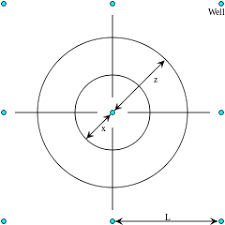The image above represents well drainage area.

To compute for the well drainage area, three essential param xeters are needed and these parameters are Total Area of Field (AT), Well Flow Rate (qwand Field Flow Rate (qr).

The formula for calculating the well drainage area:

Aw = Ar[qw / qr]

Where;

Aw = Well Drainage Area
AT = Total Area of Field
qw = Well Flow Rate
qr = Field Flow Rate

Let’s solve an example;
Find the well drainage area when the total area of field is 11, well flow rate is 9 and the field flow rate is 6.

This implies that;

AT = Total Area of Field = 11
qw = Well Flow Rate = 9
qr = Field Flow Rate = 6

Aw = AT[qw / qr]
Aw = 11[9/6]
Aw = 11[1.5]
Aw = 16.5

Therefore, the well drainage area is 16.5 ft².

Calculating the Total Area of Field when Well Drainage Area, Well Flow Rate and Field Flow Rate is Given.

AT = Aw (qr / qw)

Where;
AT = Total Area of Field
Aw = Well Drainage Area
qw = Well Flow Rate
qr = Field Flow Rate

Let’s solve an example;
Find the total area of field when the well drainage area is 32, well flow rate is 11 and the field flow rate is 14.

This implies that;
Aw = Well Drainage Area = 32
qw = Well Flow Rate = 11
qr = Field Flow Rate = 14

AT = Aw (qr / qw)
AT = 32 (14 / 11)
AT = 32 (1.272)
AT = 40.704

Therefore, the total area of field is 40.704.

Calculating the Field Flow Rate when Well Drainage Area, Total Area of Field and Well Flow Rate is Given.

qr = qw (Ar / Aw)

Where;
qr = Field Flow Rate
AT = Total Area of Field
Aw = Well Drainage Area
qw = Well Flow Rate

Let’s solve an example;
Find the field flow rate when the well drainage area is 42, total area of field is 32 and the well flow rate is 24.

This implies that;
Aw = Well Drainage Area = 42
AT = Total Area of Field = 32
qw = Well Flow Rate = 24

qr = qw (AT / Aw)
qr = 24 (32 / 42)
qr = 24 (0.7619)
qr = 18.285

Therefore, the Field Flow Rate is 18.285.

Calculating the Well Flow Rate when Well Drainage Area, Total Area of Field and Field Flow Rate is Given.

qw = qr (Aw / AT)

Where;
qw = Well Flow Rate
qr = Field Flow Rate
Aw = Well Drainage Area
AT = Total Area of Field

Let’s solve an example;
Find the well flow rate when the well drainage area is 45, total area of field is 38 and the field flow rate is 44.

This implies that;
qr = Field Flow Rate = 44
Aw = Well Drainage Area = 45
AT = Total Area of Field = 38

qw = qr (Aw / AT)
qw = 44 (45 / 38)
qw = 44 (1.184)
qw = 52.105

Therefore, the well flow rate is 52.105.

Nickzom Calculator – The Calculator Encyclopedia is capable of calculating the well drainage area

To get the answer and workings of the well drainage area using the Nickzom Calculator – The Calculator Encyclopedia. First, you need to obtain the app.

You can get this app via any of these means:

To get access to the professional version via web, you need to register and subscribe for NGN 1,500 per annum to have utter access to all functionalities.
You can also try the demo version via https://www.nickzom.org/calculator

Android (Paid) – https://play.google.com/store/apps/details?id=org.nickzom.nickzomcalculator
Android (Free) – https://play.google.com/store/apps/details?id=com.nickzom.nickzomcalculator
Apple (Paid) – https://itunes.apple.com/us/app/nickzom-calculator/id1331162702?mt=8
Once, you have obtained the calculator encyclopedia app, proceed to the Calculator Map, then click on Petroleum under EngineeringNow, Click on Reservoir Fluid Flow under Petroleum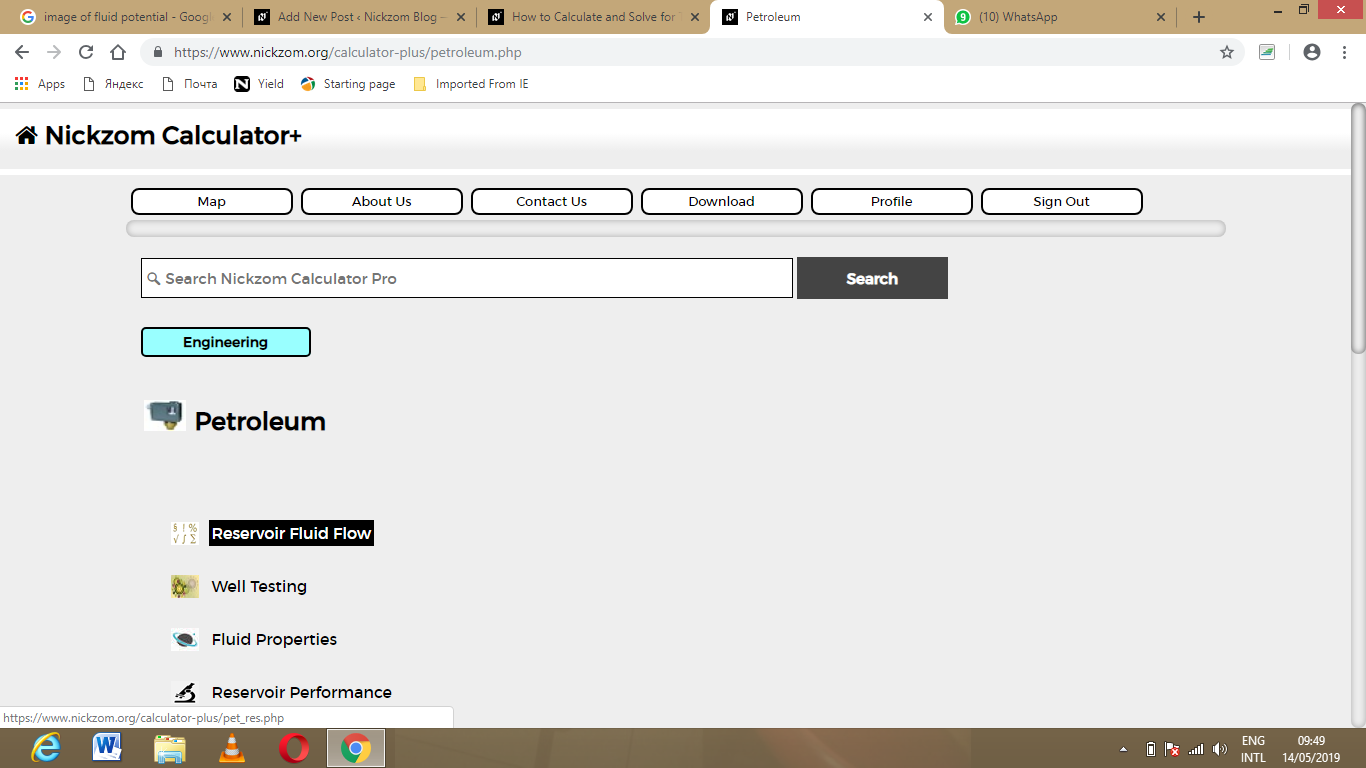Now, Click on Well Drainage Area under Reservoir Fluid Flow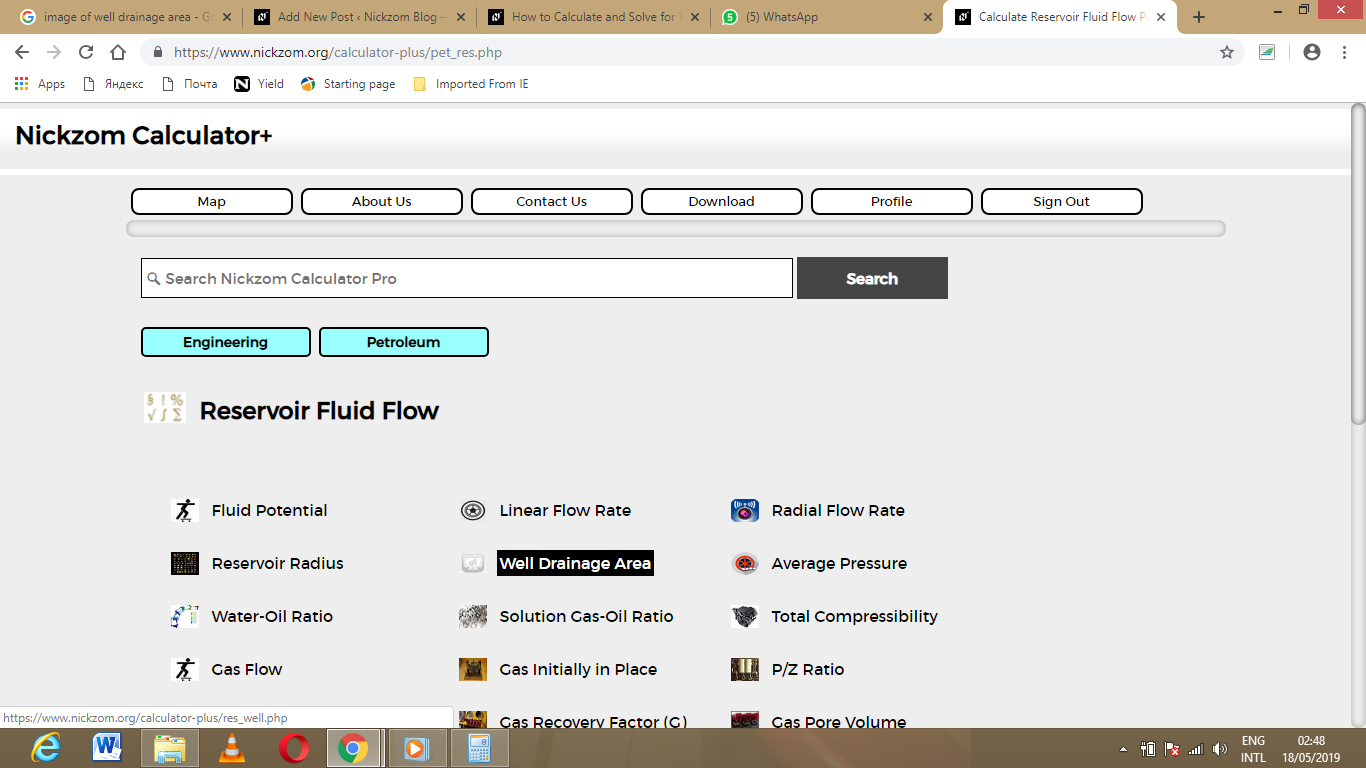The screenshot below displays the page or activity to enter your value, to get the answer for the well drainage area according to the respective parameter which are the Total Area of Field (AT), Well Flow Rate (qwand Field Flow Rate (qr).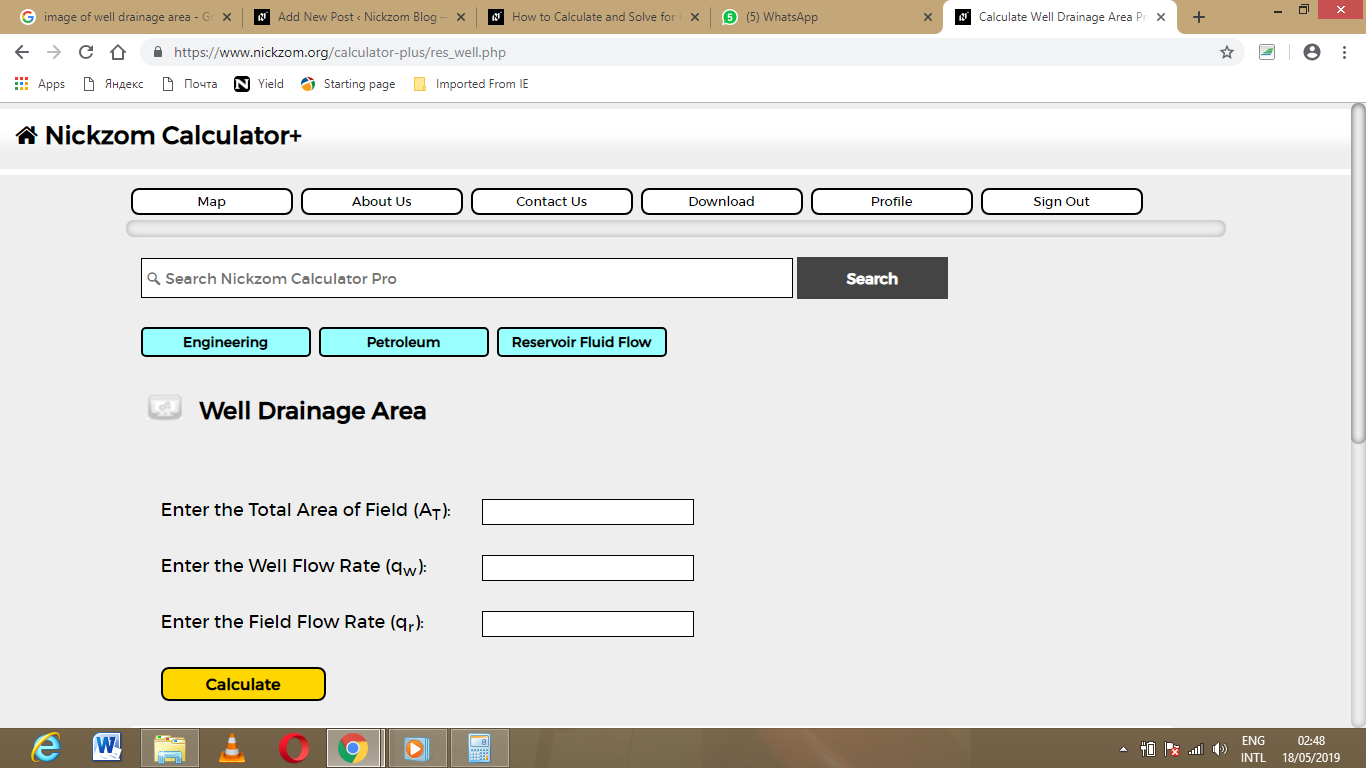Now, enter the value appropriately and accordingly for the parameter as required by the Total Area of Field (AT) is 11, Well Flow Rate (qw) is 9 and Field Flow Rate (qris 6.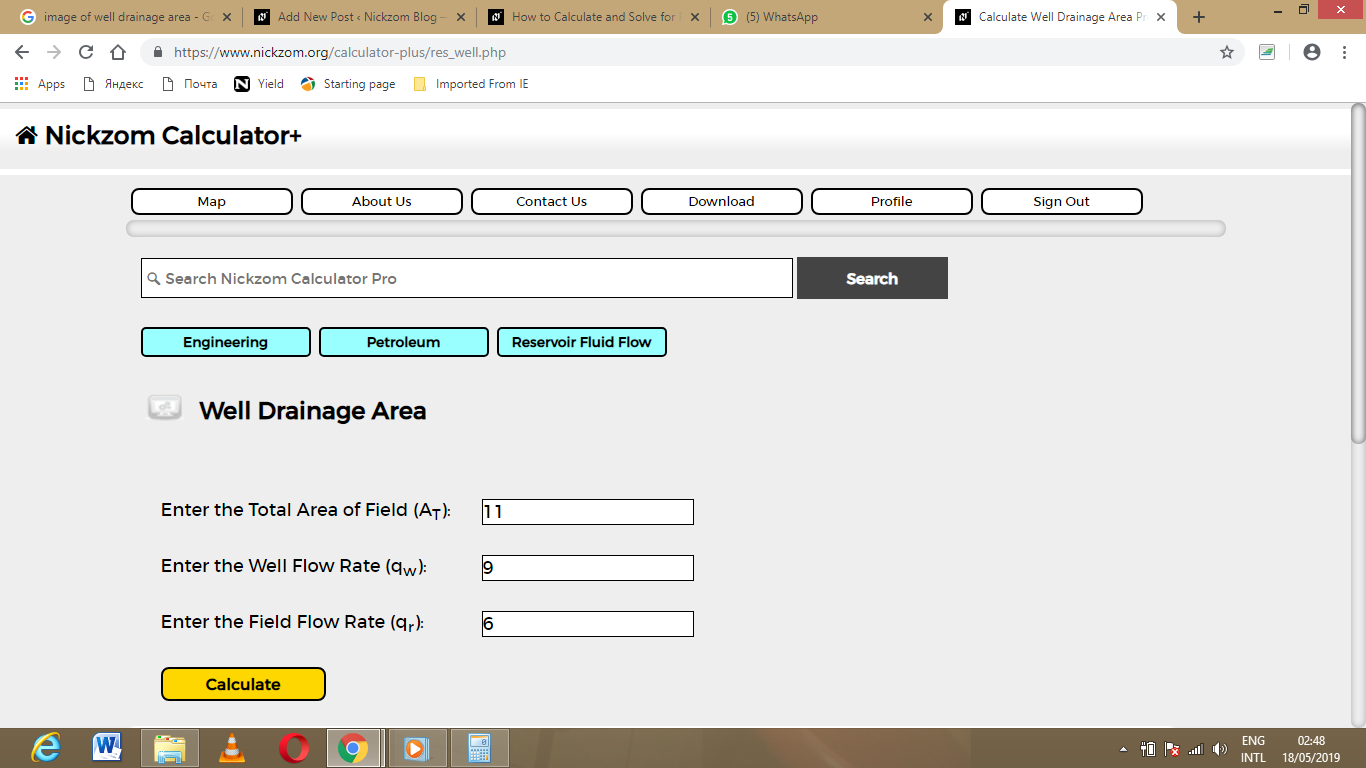Finally, Click on Calculate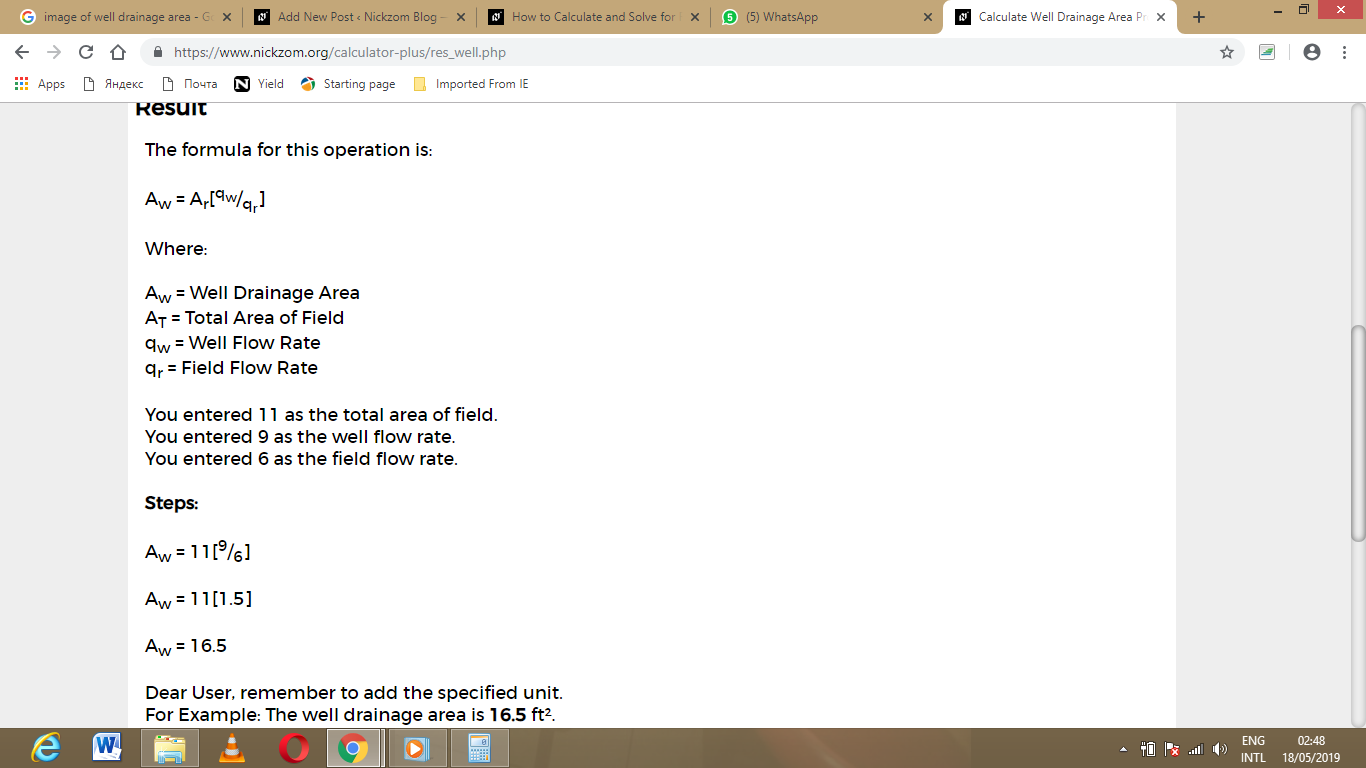As you can see from the screenshot above, Nickzom Calculator – The Calculator Encyclopedia solves for the well drainage area and presents the formula, workings and steps too.